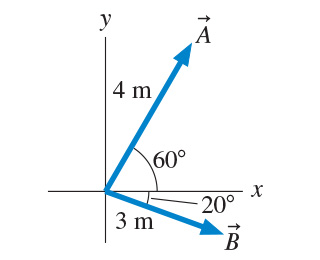# Problem: Find vector C?  such that A? +B? +C? =0? . Write your answer in component form.Express your answer in terms of the unit vectors i^ and j^. Use the 'unit vector' button to denote unit vectors in your answer. Express the coefficients using two significant figures.

###### FREE Expert Solution

The x-component of vector A  is:

Ax = Acos(60°)

The y-component of A is:

Ay = Asin(60°)

Therefore, Vector A is:

A = Acos(60°)i +  Asin(60°)j

A = (4)cos(60°)i +  (4)sin(60°)j = 2i + 3.46 j

92% (495 ratings)###### Problem Details

Find vector C?  such that A? +B? +C? =0? . Write your answer in component form.

Express your answer in terms of the unit vectors i^ and j^. Use the 'unit vector' button to denote unit vectors in your answer. Express the coefficients using two significant figures.